# HNN-extension

(diff) ← Older revision | Latest revision (diff) | Newer revision → (diff)

In 1949, G. Higman, B.H. Neumann and H. Neumann [a4] proved several famous embedding theorems for groups using a construction later called the HNN-extension. The theory of HNN-groups is central to geometric and combinatorial group theory and should be viewed in parallel with amalgamated products (cf. also Amalgam of groups).

The easiest way to define an HNN-group is in terms of presentations of groups.

## Presentation of groups.

A presentation of a groupis a pairwhereis a subset of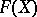, the free group on the set, andis isomorphic (cf. also Isomorphism) to the quotient group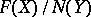, where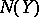is the intersection of all normal subgroups ofcontaining(cf. also Normal subgroup). The subgroupis called the normal closure ofin. See also Presentation.

Given an arbitrary group, there is an obvious homomorphismsuch that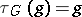for all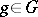. Clearly,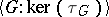is a presentation for.

## HNN-extensions.

Suppose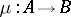is an isomorphism of subgroups of a groupandis not in. The HNN-extension ofwith respect tohas presentation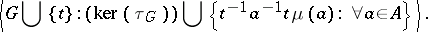The generatoris called the stable letter,the base group andandthe associated subgroups of this HNN-extension. When, the HNN-extension is called ascending.

Shorthand notation for the above group isor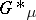.

In [a4] it was shown that the mapping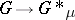taking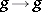for allis a monomorphism. The rest of the normal form theorem for HNN-extensions was proved by J.L. Britton in 1963 [a1] (Britton's lemma): Let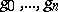be a sequence of elements ofand let the letter, with or without subscripts, denote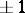. A sequence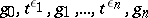will be called reduced if there is no consecutive subsequence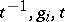with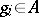or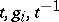with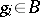. For a reduced sequence and, the elementof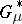is different from the unit element.

In the original reference [a4], the following theorem is proved: Every groupcan be embedded in a group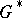in which all elements of the same order are conjugate (cf. also Conjugate elements). In particular, every torsion-free group can be embedded in a group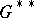with only two conjugacy classes. Ifis countable, so is. Also, every countable groupcan be embedded in a groupgenerated by two elements of infinite order. The grouphas an element of finite orderif and only ifdoes. Ifis finitely presentable, then so is.

For an excellent account of the history of HNN-extensions, see [a2]. See [a5], Chap. IV, for basic results and landmark uses of HNN-extensions, such as: the torsion theorem for HNN-extensions; the Collins conjugacy theorem for HNN-extensions; the construction of finitely-presented non-Hopfian groups (in particular, the Baumslag–Solitar group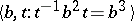is non-Hopfian; cf. also Non-Hopf group); decompositions of-relator groups; Stallings' classification of finitely-generated groups with more than one end in terms of amalgamated products and HNN-extensions; and Stallings' characterization of bipolar structures on groups.

HNN-extensions are of central importance in, e.g., the modern version of the Van Kampen theorem (based on topological results in [a8], [a9]); the Bass–Serre theory of groups acting on trees and the theory of graphs of groups (see [a7]); Dunwoody's accessibility theorem [a3]; and JSJ decompositions of groups [a6].

How to Cite This Entry:
HNN-extension. Encyclopedia of Mathematics. URL: http://encyclopediaofmath.org/index.php?title=HNN-extension&oldid=17603
This article was adapted from an original article by Mike Mihalik (originator), which appeared in Encyclopedia of Mathematics - ISBN 1402006098. See original article